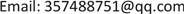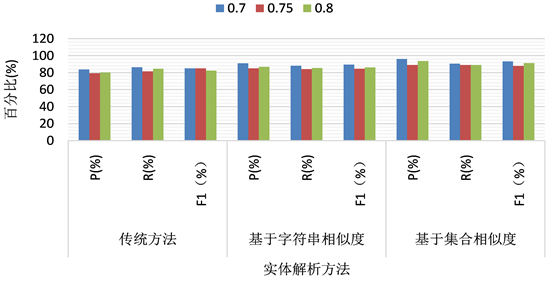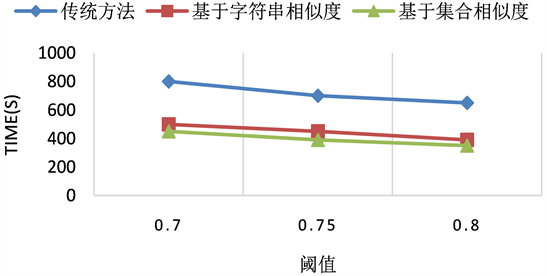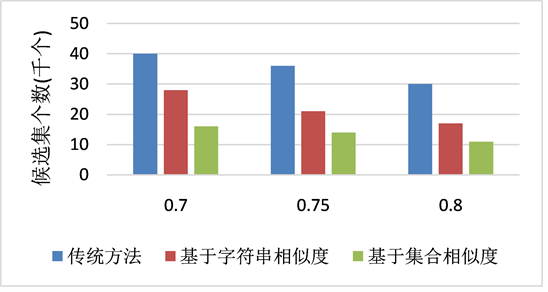﻿ 半结构化实体解析算法 Semi-Structured Entity Resolution Algorithm

Hans Journal of Data Mining
Vol. 10  No. 01 ( 2020 ), Article ID: 33406 , 15 pages
10.12677/HJDM.2020.101001

Semi-Structured Entity Resolution Algorithm

Hailang Wei, Gui Li, Zhengyu Li, Ziyang Han, Keyan Cao

School of Information and Control Engineering, Shenyang Jianzhu University, Shenyang LiaoningReceived: Nov. 21st, 2019; accepted: Dec. 4th, 2019; published: Dec. 11th, 2019ABSTRACT

Entity resolution is the identification of similar or identical records in one or more datasets. In this paper, an entity resolution algorithm based on string similarity is proposed for semi-structured data with unknown patterns. The records are divided into several substrings, and the correlation between substrings is calculated by editing similarity. On this basis, the maximum weighted matching algorithm of binary graph is introduced to measure the correlation between records. Due to the computing time complexity of this method is higher, for Web entity resolution large data sets, the time cost is larger; therefore, this article also puts forward a kind of entity resolution algorithm based on set similarity, considering record as a collection of all the property values, each attribute value as the elements in the collection, using an array of tag to represent each element, according to these tags array for each record to create a signature, to find other similar records match the signature. The optimized maximum matching algorithm is used to select the truly similar records. Finally, this paper uses the actual data set to verify that the above method is more effective than the traditional method.

Keywords:Entity Resolution, Edit Similarity, Set Similarity, Maximum Weighted Matching of Binary Graph1. 引言

1) 提出一种不考虑模式、类型的记录相似度定义，能够有效的表达半结构化记录的相似性。

2) 在本文定义的字符串相似度基础上，引入最大二分图加权匹配算法(Kuhn-Munkres算法)有效计算记录之间的相关性。

3) 为了减少候选记录之间的比较次数，引入并改进集合相似性，为记录创建有效签名空间，以及生成最优签名的步骤，将签名和倒排索引结合，对相关记录进行初步选择。

4) 为了降低最大匹配算法的时间复杂度，采用两种新的过滤器，减少不相关的候选集，同时采用三角不等式来优化最大匹配方法。

2. 相关工作

3. 相关理论

3.1. 字符串相似度

$NEds\left(x,y\right)=1-\frac{ED\left(x,y\right)}{\mathrm{max}\left(|x|,|y|\right)}$

$C\left(S\right)={\sum }_{i=1}^{k}C\left(ei\right)$

$ED\left(x,y\right)=\mathrm{min}\left\{C\left(S\right)\right\}$

3.2. 集合相关性

${\Phi }_{\alpha }\left(x,y\right)=\left\{\begin{array}{l}\Phi \left(x,y\right)\text{\hspace{0.17em}}\text{\hspace{0.17em}}\text{\hspace{0.17em}}\text{if}\text{\hspace{0.17em}}\Phi \left(x,y\right)\ge \alpha \\ 0\text{\hspace{0.17em}}\text{\hspace{0.17em}}\text{\hspace{0.17em}}\text{\hspace{0.17em}}\text{\hspace{0.17em}}\text{\hspace{0.17em}}\text{\hspace{0.17em}}\text{\hspace{0.17em}}\text{\hspace{0.17em}}\text{\hspace{0.17em}}\text{\hspace{0.17em}}\text{ }\text{ }\text{if}\text{\hspace{0.17em}}\Phi \left(x,y\right)\le \alpha \end{array}$

1) SET-SIMILARITY：检查两个集合是否相似。则SET-SIMILARITY计算公式为：

$relate{d}_{\Phi }\left(R,S\right)=simila{r}_{{\Phi }_{\alpha }}\left(R,S\right)=\frac{|R{\stackrel{˜}{\cap }}_{{\Phi }_{\alpha }}S|}{|R|+|S|-|R{\stackrel{˜}{\cap }}_{{\Phi }_{\alpha }}S|}$

2) SET-CONTAINMENT：检查一个集合是否是另一个集合的子集。其中 $|R|\le |S|$ ，则SET-CONTAINMENT的计算公式为：

$relate{d}_{\Phi }\left(R,S\right)=contai{n}_{{\Phi }_{\alpha }}\left(R,S\right)=\frac{|R{\stackrel{˜}{\cap }}_{{\Phi }_{\alpha }}S|}{|R|}$

4. 基于字符串相似度的实体解析算法

4.1. 数据分词

4.2. 记录相关性计算

${E}^{\prime }\subset E$。则记录ri和rj的相似度定义为： $Sim\left({r}_{i},{r}_{j}\right)=\frac{{\sum }_{e\in {E}^{\prime }}W\left(e\right)}{\mathrm{max}\left\{m,n\right\}}$ 其中m、n分别代表ri和rj的子字符串个数。

1) 由算法1构造两条记录的完全二分图；

2) 算法2计算两条记录相似性，其中 $similarity\left({r}_{1}\left[i\right],{r}_{2}\left[j\right]\right)$ 返回r1的第i个字符串和r2的第j个字符串之间的相似性， $optimal\text{_}match\left(B,w,m\right)$ 指的是二分图的最优匹配，返回最优匹配中所有权值之和，其中B表示完全二分图，w是B的权值矩阵，m是w的阶；

3) 根据用户需求给定阈值 $\delta$ ，用算法3将记录之间相似度大于该阈值的记录选择出来作为相似记录对。

5. 基于集合相关性的实体解析算法

5.1. 属性值规范化标记分词及创建倒排索引表Table 4. Semi-structured tag array collection representation

5.2. 有效签名生成

5.2.1. 有效签名

5.2.2. 加权签名方案

$contai{n}_{\varnothing }\left(R,S\right)=\frac{|R{\stackrel{˜}{\cap }}_{\varnothing }S|}{|R|}\ge \delta$

$|R{\stackrel{˜}{\cap }}_{\varnothing }S|\ge \delta |R|$。因此本文为 $contai{n}_{\varnothing }$ 定义了最大匹配阈值 $\theta =\delta |R|$

$simila{r}_{\varnothing }\left(R,S\right)=\frac{|R{\stackrel{˜}{\cap }}_{\varnothing }S|}{|R|+|S|-|R{\stackrel{˜}{\cap }}_{\varnothing }S|}\ge \delta$

$\delta \le \frac{|R{\stackrel{˜}{\cap }}_{\varnothing }S|}{|R|+|S|-|R{\stackrel{˜}{\cap }}_{\varnothing }S|}\le \frac{|R{\stackrel{˜}{\cap }}_{\varnothing }S|}{|R|+|S|-|S|}=\frac{|R{\stackrel{˜}{\cap }}_{\varnothing }S|}{|R|}$

${\sum }_{i=1}^{n}\frac{|{r}_{i}|-|{k}_{i}|}{|{r}_{i}|}=\frac{6-1}{6}+\frac{6-2}{6}+\frac{5-2}{5}=2.1<\theta$

5.2.3. 最优签名选择

${\sum }_{t\in {K}_{R}^{T}}|I\left[t\right]|$ 最小化， $|I\left[t\right]|$ 指的是倒排索引表中标记的个数。

1) 给出一个集合 $R=\left\{{r}_{1},{r}_{2},\cdots ,{r}_{n}\right\}$ ，对于每一个在 ${R}^{T}$ 中的t，给它赋值 $\text{value}={\sum }_{{r}_{i}|t\in {r}_{i}}\frac{1}{|{r}_{i}|}$ 以及一个代价 $\text{cost}=|I\left[t\right]|$

2) 为了最小化倒排序列的大小，在 ${R}^{T}$ 中按cost/value对所有标记以递增次序进行排序；

3) 依次选择标记，直到定义7中的条件得到满足为止，得到有效签名，其中所选的标记是 ${K}_{R}^{T}$ 的签名标记；

5.3. 候选集过滤

5.3.1. 检查过滤器

5.3.2. 最近邻过滤器

$|R{\stackrel{˜}{\cap }}_{\varnothing }S|\le {\sum }_{r\in R}\underset{s\in S}{\mathrm{max}}\Phi \left(r,s\right)$

1) 搜索有效最近邻：遍历每个标记 $t\in r$ ，对于每一个标记t，使用倒排索引表I[t]获取S中包含标记t的所有元素s。计算得到r和每个s之间的相似性；相似度得分最大的s被认为是最近邻。

2) 计算重用：在检查过滤器中，已经计算了r和 $s\in S$ 中包含r的签名标记的所有元素之间的实际相

3) 提前终止：对于 $r\in R$ 中签名标记出现在候选集合S中的元素，其最近邻相似度并不能保证

$\Phi \left(r,s\right)\le \frac{|r|-|k|}{|r|}$ ，因此要么重用检查过滤器中的计算公式，要么直接进行最近邻搜索。而对于签名标记没有出现在S集合中的元素 ${r}^{\prime }\in R$ ，对所有 $s\in S$ 边界 $\frac{|{r}^{\prime }|-|{k}^{\prime }|}{|{r}^{\prime }|}$ 仍然保留。该算法首先通过对匹配元素r的最近邻相似性进行求和来推断出一个总的估计值，并使用 $\frac{|{r}^{\prime }|-|{k}^{\prime }|}{|{r}^{\prime }|}$ 估计非匹配元素 ${r}^{\prime }$。然后，遍历每个 ${r}^{\prime }$ ，使用 ${r}^{\prime }$ 的最近邻相似性来更新总估计值。如果总估计低于θ，则删除该候选集。

5.4. 最大匹配验证

$\varphi \left(r,{s}^{\prime }\right)+\varphi \left({r}^{\prime },s\right)=1-\psi \left(r,{s}^{\prime }\right)+1-\psi \left({r}^{\prime },s\right)$ (1)

$=2-\psi \left(r,{s}^{\prime }\right)-\psi \left({r}^{\prime },r\right)$ (2)

$\le 2-\psi \left({r}^{\prime },{s}^{\prime }\right)$ (3)

$=1+1-\psi \left({r}^{\prime },{s}^{\prime }\right)$ (4)

$=\varphi \left(r,s\right)+\varphi \left({r}^{\prime },{s}^{\prime }\right)$ (5)

6. 实验评估

6.1. 数据集Table 5. Statistics of information extracted from real estate

6.2. 实验方法

6.3. 实验结果及分析Figure 1. Experimental results of the three methodsFigure 2. Running time comparison of three methods under different thresholdsFigure 3. Comparison of the number of candidate sets of the three methods

7. 总结

Semi-Structured Entity Resolution Algorithm[J]. 数据挖掘, 2020, 10(01): 1-15. https://doi.org/10.12677/HJDM.2020.101001

1. 1. Galil, Z. (1986) Efficient Algorithms for Finding Maximum Matching in Graphs. ACM Computing Surveys, 18, 23-38.
https://doi.org/10.1145/6462.6502

2. 2. Elmagarmid, A.K. and Member, S. (2007) Duplicate Record Detection: A Survey. IEEE Transactions on Knowledge and Data Engineering, 19, 1-16.
https://doi.org/10.1109/TKDE.2007.250581

3. 3. 高广尚. 面向实体解析的无监督聚类方法综述[J]. 计算机工程与应用, 2018(7): 11-19.

4. 4. 高广尚, 张智雄. 关于实体解析基本方法的研究和述评[J]. 数据分析与知识发现, 2019, 3(5): 27-40.

5. 5. 王宁, 李杰. 大数据环境下用于实体解析的两层相关性聚类方法[J]. 计算机研究与发展, 2014, 51(9): 2108-2116.

6. 6. Hernandez, M. and Stolfo, S. (1995) The Merge Purge Problem for Large Databases. ACM, New York.
https://doi.org/10.1145/223784.223807

7. 7. Monge, A.E. and Elkan, C.E. (1997) An Efficient Do-main-Independent Algorithm for Detecting Approximately Duplicate Database Records. Proceedings of Workshop on Research Issues on Data Mining and Knowledge Discovery, Newport Beach, 14-17 August 1997, 23-29.

8. 8. Gravano, L. and Ipeirotis, P.G. (2001) Using Q-Grams in a DBMS for Approximate String Processing. IEEE Data Engineering Bulletin, 24, 28-34.

9. 9. Ristad, E.S. and Yianilos, P.N. (1998) Learning String-Edit Distance. IEEE Transactions on Pattern Analysis and Machine Intelligence, 20, 522-532.
https://doi.org/10.1109/34.682181

10. 10. Deng, D., Fer-nandez, R.C., Abedjan, Z., Wang, S., Stonebraker, M., Elmagarmid, A., Ilyas, I.F., Madden, S., Ouzzani, M. and Tang, N. (2017) The Data Civilizer System. 8th Biennial Conference on Innovative Data Systems Research, Chaminade, CA, USA, 8-11 January 2017, 7 p.

11. 11. Deng, D., Li, G. and Feng, J. (2014) A Pivotal Prefix Based Filtering Algorithm for String Similarity Search. ACM SIGMOD International Conference on Management of Data, Snowbird, 22-27 June 2014, 673-684.
https://doi.org/10.1145/2588555.2593675

12. 12. Fredman, M.L. and Tarjan, R.E. (1987) Fibonacci Heaps and Their Uses in Improved Network Optimization Algorithms. Journal of the ACM, 34, 596-615.
https://doi.org/10.1145/28869.28874

13. 13. Wang, J., Li, G. and Feng, J. (2011) Fast-Join: An Efficient Method for Fuzzy Token Matching Based String Similarity Join. 27th International Conference on Data Engineering, 11-16 April 2011, 458-469.
https://doi.org/10.1109/ICDE.2011.5767865

14. 14. Wang, J., Li, G. and Feng, J. (2014) Extending String Similarity Join to Tolerant Fuzzy Token Matching. ACM Transactions on Database Systems, 39, 7.
https://doi.org/10.1145/2535628